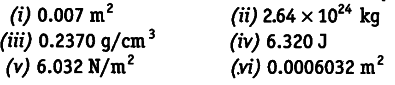# State the number of significant figures in the following

State the number of significant figures in the following.The number of significant figures in the given quantities . are given below.
(i) In 0.007, the number of significant figures is 1
because in a number less than 1, the zero’s on the right of the decimal point but to the let of the first non-zero digit are not significant.

(ii) In 2.64 X\${{10}^{24}}\$, the number of significant figures is 3. because all non-zero digits are significant, power of 10 are not taken in significant figure.
(iii) In 0.2370, the number of significant figures is 4, as all non-zero digits left to decimal and trailing zero are significant.
(iv) In 6.320, the number of significant figures is 4 (reason is same as in part ‘c ’)
(v) In 6.032, the number of significant figures is 4 (reason is same as in part c: ')
(vi) In 0.0006032, the number of significant figures is 4 (reason is same as in part ‘a’).